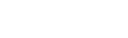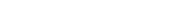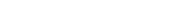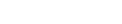How Cheenta works to ensure student success?
Explore the Back-Story

# Test of Mathematics Solution Subjective 62 - System of EquationsThis is a Test of Mathematics Solution Subjective 62 (from ISI Entrance).

The book, Test of Mathematics at 10+2 Level is Published by East West Press. This problem book is indispensable for the preparation of I.S.I. B.Stat and B.Math Entrance.

Also visit: I.S.I. & C.M.I. Entrance Course of Cheenta

## Problem

Consider the system of equations x + y = 2, ax + y = b. Find conditions on a and b under which
(i) the system has exactly one solution;
(ii) the system has no solution;
(iii) the system has more than one solution.

## Solution

### Key Idea

Solution to the linear equationsimplies there is a unique solution.implies there is either no solution or infinitely many solution.

No solution if:; infinitely many solutions other wise.

iThis is a Test of Mathematics Solution Subjective 62 (from ISI Entrance).

The book, Test of Mathematics at 10+2 Level is Published by East West Press. This problem book is indispensable for the preparation of I.S.I. B.Stat and B.Math Entrance.

Also visit: I.S.I. & C.M.I. Entrance Course of Cheenta

## Problem

Consider the system of equations x + y = 2, ax + y = b. Find conditions on a and b under which
(i) the system has exactly one solution;
(ii) the system has no solution;
(iii) the system has more than one solution.

## Solution

### Key Idea

Solution to the linear equationsimplies there is a unique solution.implies there is either no solution or infinitely many solution.

No solution if:; infinitely many solutions other wise.

i

### Knowledge Partner